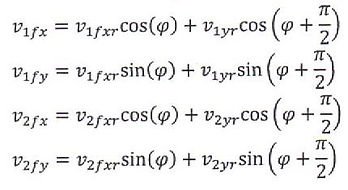Re-calculate x and y components based of a rotated axis, having the x axis parallel to the contact angle.Now solve the x components of the velocity as if they were in one dimension using the equation;Now find the x and y values for the un-rotated axis by equating for the values when the axis are rotated back.Plugging in the other equations, we get: